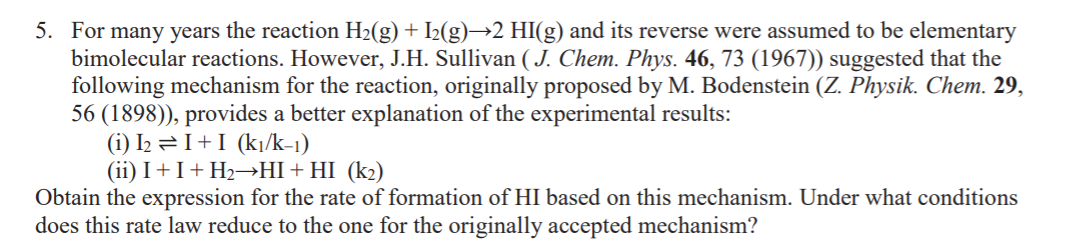# Question Solved1 Answer5. For many years the reaction H2(g) + 12(g)—2 HI(g) and its reverse were assumed to be elementary bimolecular reactions. However, J.H. Sullivan (J. Chem. Phys. 46, 73 (1967)) suggested that the following mechanism for the reaction, originally proposed by M. Bodenstein (Z. Physik. Chem. 29, 56 (1898)), provides a better explanation of the experimental results: (i) 12 = I+I (kł/k-1) (ii) I+I+H2HI+HI (k2) Obtain the expression for the rate of formation of HI based on this mechanism. Under what conditions does this rate law reduce to the one for the originally accepted mechanism?Transcribed Image Text: 5. For many years the reaction H2(g) + 12(g)—2 HI(g) and its reverse were assumed to be elementary bimolecular reactions. However, J.H. Sullivan (J. Chem. Phys. 46, 73 (1967)) suggested that the following mechanism for the reaction, originally proposed by M. Bodenstein (Z. Physik. Chem. 29, 56 (1898)), provides a better explanation of the experimental results: (i) 12 = I+I (kł/k-1) (ii) I+I+H2HI+HI (k2) Obtain the expression for the rate of formation of HI based on this mechanism. Under what conditions does this rate law reduce to the one for the originally accepted mechanism?
More
Transcribed Image Text: 5. For many years the reaction H2(g) + 12(g)—2 HI(g) and its reverse were assumed to be elementary bimolecular reactions. However, J.H. Sullivan (J. Chem. Phys. 46, 73 (1967)) suggested that the following mechanism for the reaction, originally proposed by M. Bodenstein (Z. Physik. Chem. 29, 56 (1898)), provides a better explanation of the experimental results: (i) 12 = I+I (kł/k-1) (ii) I+I+H2HI+HI (k2) Obtain the expression for the rate of formation of HI based on this mechanism. Under what conditions does this rate law reduce to the one for the originally accepted mechanism?